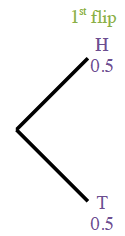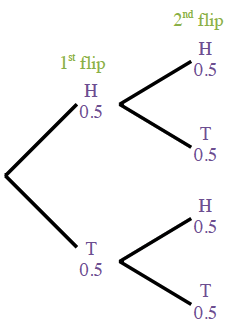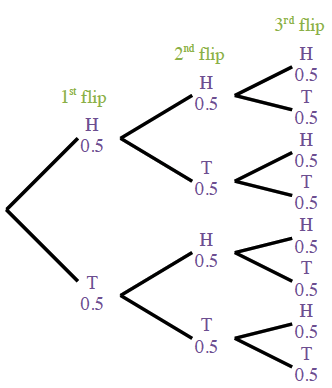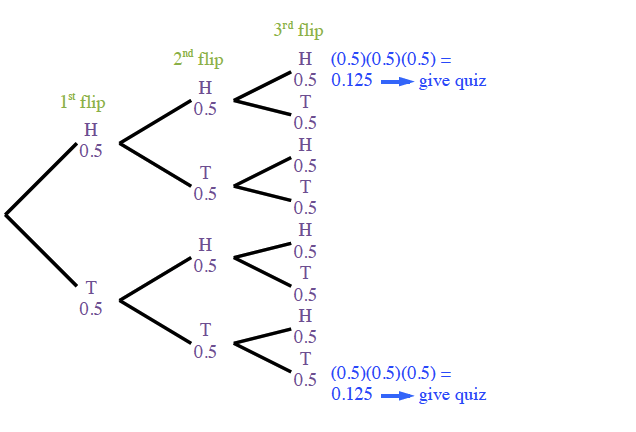### Home > CCG > Chapter 8 > Lesson 8.1.5 > Problem8-65

8-65.

Multiple Choice: To decide if his class would take a quiz today, Mr. Chiu will flip a coin three times. If all three results are heads or all three results are tails, he will give the quiz. Otherwise, his students will not be tested. What is the probability that his class will take the quiz?

1. $\frac { 1 } { 8 }$

1. $\frac { 1 } { 4 }$

1. $\frac { 1 } { 2 }$

1. $1$

Use a tree diagram to calculate the probability.

For help with the probability models, see Lesson 4.2.3.

Draw the tree diagram one step at a time.

Tree diagram titled 1st flip, with two branches: H, 0.5 and T, 0.5.

Added to each branch, 2 branches titled 2nd flip, top branch labeled H & 0.5, & bottom branch labeled, T & 0.5, on each of the 2 pairs.

Added to each of those branches, 2 branches titled, 3rd flip, top branch labeled H & 0.5, & bottom branch labeled, T & 0.5, on each of the 4 pairs.

Calculate the probability of three consecutive heads (H) or tails (T).

If you follow the branches of the tree diagram for H, H, H you get the P(H,H,H) by multiplying $(0.5)(0.5)(0.5) = 0.125$ --> give quiz

If you follow the branches of the tree diagram for T, T, T you get the P(T,T,T) by multiplying $(0.5)(0.5)(0.5) = 0.125$ --> give quiz

Calculate the probability that the teacher will give the quiz. To do so add the P(H,H,H) + P(T,T,T).

P(give quiz) = $0.125 + 0.125 = 0.25$.

B; see response in Step 5 for reason.